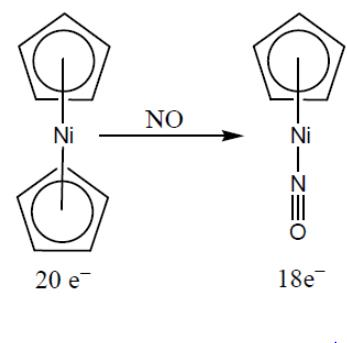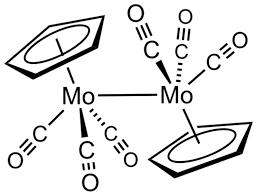Courses

# Test: Organometallic Chemistry- 1

## 20 Questions MCQ Test Inorganic Chemistry | Test: Organometallic Chemistry- 1

Description
This mock test of Test: Organometallic Chemistry- 1 for Chemistry helps you for every Chemistry entrance exam. This contains 20 Multiple Choice Questions for Chemistry Test: Organometallic Chemistry- 1 (mcq) to study with solutions a complete question bank. The solved questions answers in this Test: Organometallic Chemistry- 1 quiz give you a good mix of easy questions and tough questions. Chemistry students definitely take this Test: Organometallic Chemistry- 1 exercise for a better result in the exam. You can find other Test: Organometallic Chemistry- 1 extra questions, long questions & short questions for Chemistry on EduRev as well by searching above.
QUESTION: 1

Solution:
QUESTION: 2

### The substitution of η5-Cp group with nitric oxide is the easiest for:

Solution:QUESTION: 3

### Among the following, the unstable carbonyl species is:

Solution:
QUESTION: 4

Oxidation number of Co in [Co(NO)(CO)3] is:

Solution:
QUESTION: 5

The organometallic compound W(C5H5)2(CO)2 follows the 18-electron rule, The hapticities of the two cyclopentadienyl groups are:

Solution:

W is 6 electron species while CO will give 2 electrons

So, 1W + 2(CO) = 10 electrons.

Remaining 8 electrons will be coming from 2Cp.

Hapticity means no of electrons donated by the ligand.

Here 5 + 3 = 8. So one Cp = 5

Hapticity One Cp = 3

Hapticity Total 1 W + 2 (CO) + 1 ( Cp-5) + 1 (Cp-3)

= 6+4+5+3

= 18

QUESTION: 6

For a square planar complex [Mabcd] (where M = central metal and abcd are monodentate ligands), the number of possible geometrical isomers are:

Solution:
QUESTION: 7

Which of the following will exhibit optical isomerism:

Solution:
QUESTION: 8

The compounds [PtCl2(NH3)4]Br2 and [PtBr2(NH3)4]Cl2 constitutes a pair of:

Solution:
QUESTION: 9

The order of CO bond strengths in the following metal hexacarbonyls is likely to be:

Solution:
• In the isoelectronic series of metal carbonyl, the CO bond strength is expected to increase in the order [V(CO)-6]< [Cr(CO)6]< [Mn(CO)+6]
• The oxidation state of the central metal atom increases in the order V(−) < Cr(0) < Mn(+) .
• As the oxidation state of the central metal atom increases, less and less electron density is donated to the carbonyl ligand.
• This increases the CO bond strength as the added electron density occupies the anti-bonding orbital.
QUESTION: 10

How many M — M bonds are present in [Cp Mo(CO3)]2?

Solution:

Cyclopentadienylmolybdenum tricarbonyl dimer is the chemical compound with the formula Cp2Mo2(CO)6, where Cp is C5H5.

No. of M-M bonds = (18×2-[(6+5+2×3)×2])/2=0.9 ~ 1QUESTION: 11

Which of the following complex is in which organic ligand is having only bond with metal?

Solution:

CH3 is the only σ-donor ligand while C2H4, C5H5, C6H6 are π-acceptor ligands.

QUESTION: 12

Solid Co2(CO)8 shows infrared CO stretching bands at 1857, 1886, 2001, 2031, 2044, 2059, 2071 and 2112 cm–1. When Co2(CO)8 is dissolved in hexane, the carbonyl bands at 1857 and 1886 cm–1 disappear. These changes in the infrared spectrum in hexane are due to:

Solution:
QUESTION: 13

Which one of the following specie will be most easily reduced:

Solution:
QUESTION: 14

The final product in the reaction

[Mn (CO)6]-  + MeLi → is :

Solution:
QUESTION: 15

In the complex, [Ni2(η5-Cp)2(CO)2] the IR stretching frequency appears at 1857 cm-1 (strong) and 1897 cm-1 (weak). The valence electron count and the nature of the M—CO bond respectively are:

Solution:
QUESTION: 16

Amongst the following, the metal that does not form homoleptic polynuclear metal carbonyl is:

Solution:
QUESTION: 17

Complexes of general formula, fac–[Mo(CO)3(Phosphine)3] have the C—O stretching frequency bands as given below,
Phosphines: PF3 (P), PCl3 (Q), P(Cl)Ph2 (R), PMe3(S)
ΝCO, cm–1 : 2090 (I); 2040 (II); 1977 (III); 1945 (IV)

Solution:
QUESTION: 18

What kind of isomerism is exhibited by octahedral [Co(NH3)4Br2]Cl:

Solution:
QUESTION: 19

In which of the following pairs, both the complexes show optical isomerism:

Solution:
QUESTION: 20

The ionization isomer of [Cr(H2O)4Cl(NO2)]Cl is :

Solution:

The ionization isomer of [Cr(H2O)4Cl(NO2)]Cl is [Cr(H2O)4Cl2](NO2)